# Physics - Classical Mechanics - Elastic and Inelastic Collisions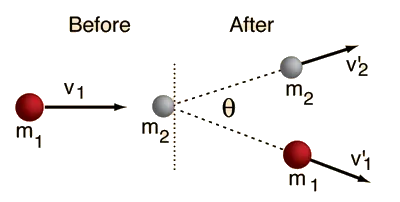[Image 1]

## Introduction

Hey it's a me again @drifter1! Today we continue with Physics and more specifically the branch "Classical Mechanics" to talk about the two types of Collisions. So, without further ado, let's get straight into it!

## Collision

Let's suppose that we have some moving objects travelling through space, which means that those objects travel with some speed/velocity v. Therefore, each of those objects has some Kinetic Energy KE and Momentum p. Supposing that the mass is m these two quantities can be calculated as following:Let's also recall two very important laws of Physics that apply to motion:
• Conservation of Energy: Energy can neither be created nor destroyed. It just can be converted from one from of energy into another.
• Conservation of Linear Momentum: The total linear momentum of a system of particles is conserved when there is no external force acting on the system. So, the total linear momentum before is equal to the total linear momentum after a collision.
A collision is defined as the "abrupt change in path of a moving body (or bodies) due to its interaction with another body (or bodies)". So, the freely moving objects, that we described previously, now interact with each other, meaning that the magnitude and direction of the velocity of them might change after a collision. The force that is involved during such collisions acts for a very short period of time. But, what's interesting about those collisions? Well, knowing the state of a system before a collision, we can calculate at which state the system will be after the collision. This will let us calculate the velocity, position etc. of the objects that took part at the collision.

But, it's not that easy! We don't have only one type of collision and that's it! Actually, there are two types of collisions:
1. Elastic Collision: In this type of collision both the momentum and kinetic energy of the system are conserved. In such collisions the forces involving are conservative in nature.
2. Inelastic Collision: In this type of collision only momentum is conserved and not kinetic energy. Most collisions in our daily life are inelastic in nature.
So, let's get into these two types on their own now!

## Elastic Collision

As we said previously both the momentum and the kinetic energy are conserved during an elastic collision. So, thinking about two objects with different mass (m1 and m2) and initial velocity (v1 and v2), to simplify the problem, we can write the following two equations which show us that these two quantities stay the same before (initial) and after (final) a collision:After solving these final equations we find that the final velocity of each object is given by the equations:
You can check out the complete proof of the equations at Khan Academy

Before we continue with inelastic collisions, let's first get into some very interesting cases of elastic collisions...

### Objects of equal mass with one at rest

In this case, the impacting object comes to a dead stop, while the target gains the exact same speed as the impacting object: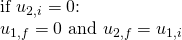### Objects of equal mass with oppositely directed velocity

A more generalized case of the previous case is the one of having objects of equal mass "move into each other". In that case the two objects bounce off each other exchanging velocity. So, we have: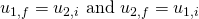### Heavy object colliding with much lighter target which is at rest

Having a heavy object collide with a very light target, the heavy object "tends to" stay at its initial velocity. This means that the collision with the light target had very little effect on the heavy object.

### Light object colliding with much heavier target which is at rest

Having a light object collide with a very heavy object, the light object bounces off the target, maintaining the same speed but with opposite direction. The heavy target remains at rest, acting like a wall.

## Inelastic Collision

Getting into inelastic collisions, we can clearly say that there is some loss of kinetic energy, due to the transformation of some of that kinetic energy into some other form of energy. This other form can be thermal energy, sound energy or material deformation. Getting to the very extreme of losing the maximum possible kinetic energy after a collision, we talk about a perfectly inelastic collision. This of course doesn't mean that the final kinetic energy is necessarily zero as the momentum still has to be conserved. One great example of such an perfectly inelastic collision is the ballistic pendulum.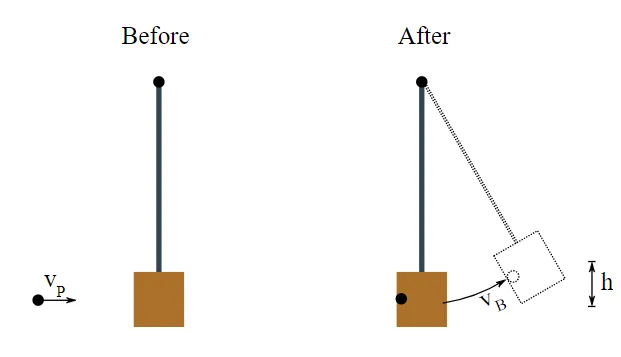[Image 2]

In this pendulum a particle P is being shot into a wooden block that's hanging on a rope. As only momentum is conserved during that collision we have: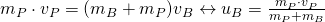Supposing that the total mechanical energy of the block-particle system is being conserved and considering that the block rises up to a maximum height h under a gravitational acceleration g, we get: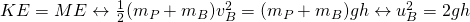Substituting the velocity into the previous expression of conservation of momentum, we can now calculate the speed of the particle: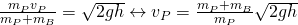## RESOURCES:

### References

1. http://hyperphysics.phy-astr.gsu.edu/hbase/elacol.html
2. http://vlab.amrita.edu/?sub=1&brch=74&sim=189&cnt=1

### Images

Mathematical equations used in this article, where made using quicklatex.

## Final words | Next up

This is actually it for today's post! Next time we will get into mathematical examples of those Collisions...

See ya!Keep on drifting!

H2
H3
H4
3 columns
2 columns
1 column